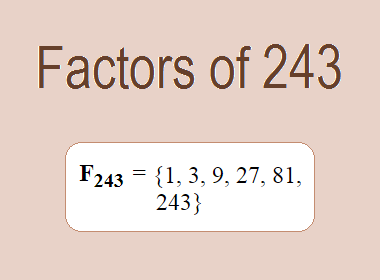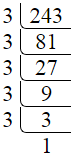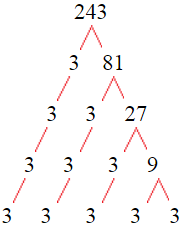# Factors of 243The factors of 243 are 1, 3, 9, 27, 81, and 243 i.e. F243 = {1, 3, 9, 27, 81, 243}. The factors of 243 are all the numbers that can divide 243 without leaving a remainder.

We can check if these numbers are factors of 243 by dividing 243 by each of them. If the result is a whole number, then the number is a factor of 243. Let's do this for each of the numbers listed above:

·        1 is a factor of 243 because 243 divided by 1 is 243.

·        3 is a factor of 243 because 243 divided by 3 is 81.

·        9 is a factor of 243 because 243 divided by 9 is 27.

·        27 is a factor of 243 because 243 divided by 27 is 9.

·        81 is a factor of 243 because 243 divided by 81 is 3.

·        243 is a factor of 243 because 243 divided by 243 is 1.

## How to Find Factors of 243?

1 and the number itself are the factors of every number. So, 1 and 243 are two factors of 243. To find the other factors of 243, we can start by dividing 243 by the numbers between 1 and 243. If we divide 243 by 2, we get a remainder of 1. Therefore, 2 is not a factor of 243. If we divide 243 by 3, we get a remainder of 0. Therefore, 3 is a factor of 243.

Next, we can check if 4 is a factor of 243. If we divide 243 by 4, we get a remainder of 3. Therefore, 4 is not a factor of 243. We can continue this process for all the possible factors of 243.

Through this process, we can find that the factors of 243 are 1, 3, 9, 27, 81, and 243. These are the only numbers that can divide 243 without leaving a remainder.

********************

********************

## Properties of the Factors of 243

The factors of 243 have some interesting properties. One of the properties is that the sum of the factors of 243 is equal to 364. We can see this by adding all the factors of 243 together:

1 + 3 + 9 + 27 + 81 + 243 = 364

Another property of the factors of 243 is that they are all odd numbers. This is because 243 is an odd number, and any even number cannot divide an odd number without leaving a remainder.

Another property of the factors of 243 is that the only prime factor of 243 is 3.

## Applications of the Factors of 243

The factors of 243 have several applications in mathematics. One of the applications is in finding the highest common factor (HCF) of two or more numbers. The HCF is the largest factor that two or more numbers have in common. For example, to find the HCF of 243 and 90, we need to find the factors of both numbers and identify the largest factor they have in common. The factors of 243 are 1, 3, 9, 27, 81, and 243. The factors of 90 are 1, 2, 3, 5, 6, 9, 10, 15, 18, 30, 45, and 90. The largest factor that they have in common is 9. Therefore, the HCF of 243 and 90 is 9.

Another application of the factors of 243 is in prime factorization. Prime factorization is the process of expressing a number as the product of its prime factors. The only prime factor of 243 is 3. We can express 243 as:

243 = 3 × 3 × 3 × 3 × 3

We can do prime factorization by division and factor tree method also. Here is the prime factorization of 243 by division method,243 = 3 × 3 × 3 × 3 × 3

Here is the prime factorization of 243 by the factor tree method,243 = 3 × 3 × 3 × 3 × 3

## Conclusion

The factors of 243 are the numbers that can divide 243 without leaving a remainder. The factors of 243 are 1, 3, 9, 27, 81, and 243. The factors of 243 have some interesting properties, such as being odd numbers and having a sum of 364. The factors of 243 have several applications in mathematics, such as finding the highest common factor and prime factorization.